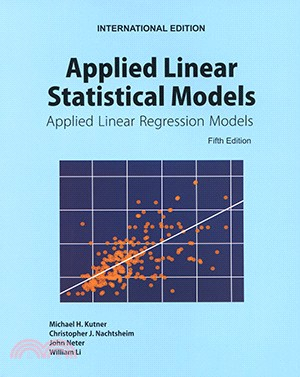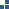瀏覽紀錄

TOP
1/1無庫存，下單後進貨(採購期約4~10個工作天)一般分類科學‧科普 > 自然科學 > 數學 > 代數
定  價：NT\$1280元
可得紅利積點：38 點

無庫存，下單後進貨(採購期約4~10個工作天)

商品簡介

作者簡介

目次

1. Added material on important techniques for data mining, including regression trees and neural network models in Chapters 11 and 13.
2. The Chapter on logistic regression (Chapter 14) has been extensively revised and expanded to include a more thorough treatment of logistic, probit, and complementary log-log models, logistic regression residuals, model selection, model assessment, logistic regression diagnostics, and goodness of fit tests. We have also developed new material on polytomous (multicategory) nominal logistic regression models and polytomous ordinal logistic regression models.
3. We have expanded the discussion of model selection methods and criteria. The Akaike information criterion and Schwarz Bayesian criterion have been added, and a greater emphasis is placed on the use of cross-validation for model selection and validation.
4. New open ended 'Cases' based on data sets from business, health care, and engineering are included. Also, many problem data sets have been updated and expanded.
5. The text includes a CD with all data sets and the Student Solutions manual in PDF. In addition a new supplement, SAS and SPSS Program Solutions by Replogle and Johnson is available for the Fifth Edition.
作者：Michael H. Kutner
現職：Emory University

作者：Christopher J. Nachtsheim
現職：University of Minnesota

作者：John Neter
現職：University of Georgia

作者：William Li
現職：University of Minnesota
PART I: SIMPLE LINEAR REGRESSION
Ch 1 Linear Regression with One Predictor Variable
Ch 2 Inferences in Regression and Correlation Analysis
Ch 3 Diagnostics and Remedial Measures
Ch 4 Simultaneous Inferences and Other Topics in Regression Analysis
Ch 5 Matrix Approach to Simple Linear Regression Analysis

PART II: MULTIPLE LINEAR REGRESSION
Ch 6 Multiple Regression I
Ch 7 Multiple Regression II
Ch 8 Regression Models for Quantitative and Qualitative Predictors
Ch 9 Building the Regression Model I: Model Selection and Validation
Ch10 Building the Regression Model II: Diagnostics
Ch11 Building the Regression Model III: Remedial Measures
Ch12 Autocorrelation in Time Series Data

PART III: NONLINEAR REGRESSION
Ch13 Introduction to Nonlinear Regression and Neural Networks
Ch14 Logistic Regression, Poisson Regression, and Generalized Linear Models

## 購物須知

為了保護您的權益，「三民網路書店」提供會員七日商品鑑賞期(收到商品為起始日)。

若要辦理退貨，請在商品鑑賞期內寄回，且商品必須是全新狀態與完整包裝(商品、附件、發票、隨貨贈品等)否則恕不接受退貨。